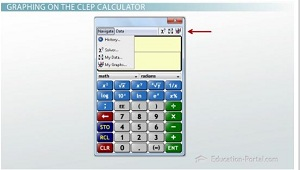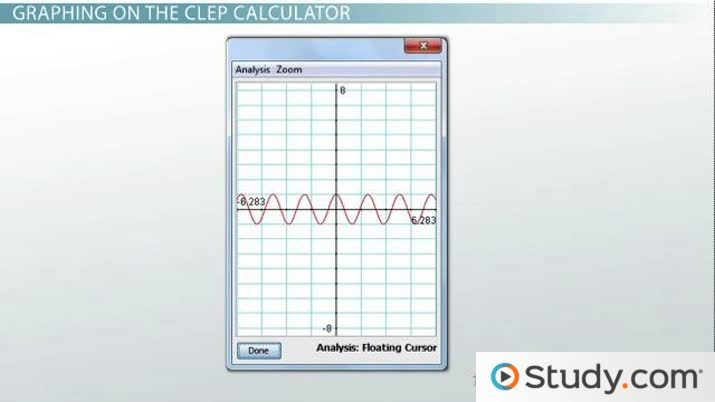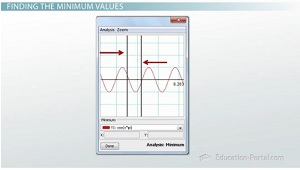How to Solve Equations by Graphing on the CLEP Scientific Calculator

Lesson Transcript
Instructor: Kelly Sjol
A CLEP calculator is useful in calculating and solving different mathematical equations such as finding the intersections of multiple functions. Learn more about solving equations by graphing on the CLEP scientific calculator. Updated: 10/27/2021

Graphing on the CLEP Calculator

Let's take a quick look at graphing on the CLEP calculator.

You can enter the graphing mode on the CLEP calculator by choosing the mode that says 'Navigate: My Graphs', or, you can click on the little 'graph' button in the upper right corner. When you enter this mode, well, it looks pretty sparse. It just says My Graphs at the top, has a big blank space and then a bunch of buttons at the bottom: 'New', 'Window', 'Clear', 'Done', 'Table' and 'Graph'. You can try to graph right now, but all you'll get is this piece of, basically, graph paper.

In order to graph a function, you have to click 'New'. When you click 'New', you're first given a choice of 'Function', 'Polar' or 'Parametric'. Usually, you'll want to choose 'Function'; in fact, for the entirety of this calculus course, you'll want to choose only 'Function'. Not 'Polar', and not 'Parametric'. So let's pick 'Function' for now.Now this window is exactly like the Solver window, y(x):, except that now you're not going to type 'y equals something,' you're just going to type what's on the right side, so 'y(x):' is just like 'y(x) equals whatever you type.'

So let's graph the cosine of x times pi. Once we've typed it in here, cos(x*pi), we're going to click 'OK'. This takes us back to the My Graphs window, except now we have y1:cos(x*pi). This also gives us a couple of other options: First, there are the checkboxes on the far left. If this box is checked, then any time we go to our graphs screen, it will show this function. If it's not checked, this function won't show up on the graph. This column here shows you the type of line that will be graphed, and this shows the color of our graph. So in this case, we expect it to be a red solid line for y=cos(x*pi).

So let's click 'Graph' and see what this looks like. Sure enough, there's our graph of cos(x*pi) as a red, solid line. Let's zoom in. I'm going to click 'Zoom', and then 'Trig'. That gives me a graph from x=(-2)pi to x=2 pi, but let's zoom in a little bit further. I'm just going to click where I want to zoom. Looks like that's a little too far; let's zoom out some. Okay.

So now we're looking at this graph, and we've got our standard, default floating cursor for this graph. Now this cursor will give you the x and y coordinates of any point on this graph. But I don't really care that this point might be 3.2 or this point might be 2 pi in the x direction and 2 in the y direction. I want to know where the value of this graph is for certain values of x. So I'm going to choose 'Analysis: Trace/Evaluate'. And now I'm going to hover over various points along this graph.

So as soon as I choose 'Trace/Evaluate', I get this bottom window that first shows me what line I'm looking at. In this case, I'm looking at the red line, which is y1, which is equal to cos(x*pi). So any point is going to be along this line. Below that, I have the x and y coordinates of the point on that line that is denoted with this x. So I can trace the line, increasing x, and watch what happens to y.An error occurred trying to load this video.

Try refreshing the page, or contact customer support.

Coming up next: Using Unit Circles to Relate Right Triangles to Sine & Cosine

You're on a roll. Keep up the good work!

Replay
Your next lesson will play in 10 seconds
• 0:06 Graphing on the CLEP…
• 4:10 Finding the 0 Value
• 5:00 Finding the Minimum Values
• 5:22 Graphing Two Equations
• 6:24 Finding Intersections
• 9:04 Lesson Summary
Save Save

Want to watch this again later?

Timeline
Autoplay
Autoplay
Speed Speed

Finding the Zero ValueThere are other options under the 'Analysis' menu, such as 'Zero', 'Minimum' and 'Maximum'. So let's click on 'Zero'. First, it says down in the bottom right-hand corner that I'm doing a zero analysis. What this does is it allows me to choose a range. So I click once to create the left side of the range, and I drag over and release to select the entire range. And what the calculator will do is it will find the first point where my function equals zero in that range. So here it's picked the point x=2.5. Obviously y=0, because I was looking for a point where y equaled zero in my range. Once you're in this analysis, you can select as many ranges as you want and find what x is for all of these zero, or root, values.

Finding the Minimum Values

Let's try finding a minimum. It's the same process here. Now I click to select one side of my range, and I drag to select the entire range. So here, the range goes from this line to this line, and the calculator will find the minimum point within that range. You can do the same thing with maximum.

To unlock this lesson you must be a Study.com Member.

Register to view this lesson

Are you a student or a teacher?